# Renormalization

(diff) ← Older revision | Latest revision (diff) | Newer revision → (diff)

A procedure for eliminating divergences occurring in perturbation theory in the Lagrange formulation of quantum field theory. In the construction of formal series in quantum field theory by perturbation theory, expressions appear that do not have an unambiguous mathematical sense; they are related to the so-called ultraviolet divergences. Such divergences arise because the coefficients in these series are products of generalized functions, i.e. the object is, in general, not well defined.

It is convenient to begin the discussion with the regularized theory, in which the generalized functions are replaced by sufficiently-smooth functions. Regularization introduces additional parameters into the theory that do not have a direct physical sense. However, in the regularized theory one may isolate from each coefficient function a part that gives rise to the ultraviolet divergence on removing the regularization. Renormalization proper consists in discarding the divergent contributions in the coefficient functions. After renormalization, the regularization is removed, i.e. the regularization parameter is eliminated by passing to the corresponding limit.

The idea of renormalization was proposed by H.A. Bethe  and consists in discarding the divergences in such a way so as to obtain a re-definition (renormalization) of the parameters in the initial Lagrangian, i.e. the bare masses, coupling constants and the field normalization. An exact formulation for the renormalization procedure in quantum field theory (theoperation) was given by N.N. Bogolyubov and O.S. Parasyuk . They proved a theorem (the Bogolyubov–Parasyuk theorem) on the finiteness of the renormalized expressions in the sense of generalized functions from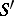in each order of perturbation. Here the renormalization amounts to adding terms ( "counterterms" ) to the Lagrangian. Each counterterm is a certain local operator having a numerical coefficient that, in general, is an infinite series in the bare coupling constants. These coefficients are finite only in the presence of regularization and become infinite when it is eliminated. Therefore, renormalization demonstrates the auxiliary character of the initial Lagrangian. Only the renormalized Lagrangian has physical significance. Its parameters, i.e. the renormalized masses, coupling constants, etc., which are finite, can be identified with observable quantities.

A Lagrangian formulation for perturbation theory is possible only for theories in which the number of counterterms differing in operator structure is finite. Such theories are divided into two classes: superrenormalizable and renormalizable. In the superrenormalizable theories, the coefficients at the counterterms are finite series in the coupling constants, whereas these series are infinite in the renormalizable theories. In these theories, the operator structures in the counterterms are usually the same as in the individual terms in the initial Lagrangian. Their union leads to the renormalization of the bare coupling constants.

In its classical form, renormalization is the Bogolyubov–Parasyukoperation (eliminating divergences from each diagram arising, for example, in the expansion of thematrix, cf. Scattering matrix) and amounts to re-defining the coefficient function for that diagram as follows. Letbe an operator that puts the coefficient function for a diagraminto correspondence with the initial segment of the expansion as a MacLaurin series in the momenta. Then the coefficient function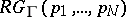for the renormalized diagramis written as(*)

where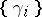is the set of all divergent subdiagrams of, while the symbol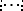means first discarding all terms containing the products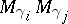corresponding to pairs of subdiagrams for which none of the conditions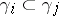,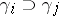,is fulfilled on expanding the parentheses in (*), and, secondly, ordering the operatorsin such a way that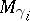stands to the right of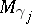if.

The restrictions imposed on the renormalization procedure do not define it completely. The remaining arbitrariness is of dual character. First, the requirement that the renormalized expressions are finite determines only a certain minimal number of "subtractions" in each divergent subdiagram, i.e. a minimal number of terms in the MacLaurin series. Renormalization will lead, in the first place, to finite results as before if the number of subtractions is increased while fulfilling certain compatibility conditions for the subtractions in the various subdiagrams of the renormalized diagram. Secondly, instead of a segment of a MacLaurin series, one may subtract an expression differing from it by a polynomial in the momenta of the same degree and having finite coefficients.

This arbitrariness leads to equivalence between renormalization procedures differing in formulation from theoperation. The concrete choice is usually dictated by the task under consideration. For example, for theories with gauge invariance a renormalization has been proposed that has been called dimensional. It is based on regularization and employs as parameter a deviation from the physical value of the space-time for which the calculation is performed. The divergences are eliminated by discarding expressions singular in this deviation.

In other cases one uses analytic renormalization, based on regularization involving the replacement of a particle propagator taking the form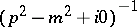by. The continuation into the complex domain with respect to the parameterprovides the regularization, while the ultraviolet divergences are distinguished as poles in certain linear combinations of these parameters. A compatible discarding of such poles eliminates all the divergences.

Other renormalization schemes are also used in applications .

How to Cite This Entry:
Renormalization. Encyclopedia of Mathematics. URL: http://encyclopediaofmath.org/index.php?title=Renormalization&oldid=14347
This article was adapted from an original article by S.A. Anikin (originator), which appeared in Encyclopedia of Mathematics - ISBN 1402006098. See original article